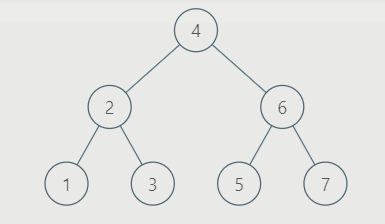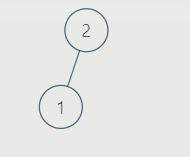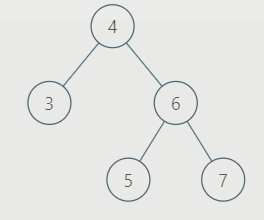# Split a BST into two balanced BSTs based on a value V

## Problem statement

Given a Binary Search Tree (BST) with a root node and a target value V, break the tree into two Balanced binary search tree such that one subtree has nodes that are all greater or equal to the target value V, while the other subtree has all nodes that are lesser than the target value V. It's not necessarily the case that the tree contains a node with value V.

Example

Input:V = 3

Output:

First BST = [2,1]

Second BST = [4,3,6,5,7]

After splitting the given BST about given value V = 3

First Balanced Binary Search Tree isSecond Balanced Binary Search Tree is## Approach

The idea here is to store the inorder traversal in the array and further use that inorder traversal to split the array about the value ‘V’, then making the balanced binary search tree from both the split parts of that array.

## Algorithm

• First make a dummy array to store the inorder traversal of BST
• Then split the dummy array about the value ‘V’ and store the split index
• Construct Balanced BST from both the split array that is from the left part of the split index and right part of the split index
• To construct the balanced BST from the sorted dummy array storing the inorder traversal follow the given steps
• Get the Middle of the array and make it root of balanced BST
• Recursively do same for left half and right half of the array to make small balanced BST and attach these to small balanced BST to the root node which was themiddle element of the array

## Code:

``````#include <bits/stdc++.h>
using namespace std;

// BST tree node structure
class TreeNode {
public:
int data;
TreeNode *left, *right;
};

//Function for creating a new node of BST
TreeNode* newNodeBST(int value)
{
TreeNode* temp = new TreeNode();
temp->data = value;
temp->left = temp->right = nullptr;
return temp;
}

//Function for inserting a new node in BST
TreeNode* insert(TreeNode* node,int value)
{

// If tree is empty make the new node and return the same
if (node == NULL)
return newNodeBST(value);

// if tree is not empty simply recur to insert the node
if (value < node->data)
node->left = insert(node->left,value);
else if (value > node->data)
node->right = insert(node->right,value);

return node;
}

// Function to return size of the tree
int treeSize(TreeNode* root)
{
if (root == NULL) {
return 0;
}

// Calculate left and right size recursively
return 1 + treeSize(root->left) + treeSize(root->right);
}

// Inorder traversal of the tree
void traversalInorder(TreeNode* root)
{
if (root == NULL)return;

traversalInorder(root->left);
cout << root->data << " ";
traversalInorder(root->right);
}

// Function to store inorder traversal
void storeInorder(TreeNode* root, int inOrder[], int& index)
{
// Base condition
if (root == NULL) {
return;
}

// Storing left and right part recursively
storeInorder(root->left, inOrder, index);
inOrder[index++] = root->data;
storeInorder(root->right, inOrder, index);
}

// Returning the splitting index of array
int getSplittingIndex(int inOrder[], int index, int V)
{
for (int i = 0; i < index; i++) {
if (inOrder[i] >= V) {
return i - 1;
}
}
return index - 1;
}

// Balance BST formation
TreeNode* createBST(int inOrder[], int start, int end)
{

// Base Condition
if (start > end) {
return NULL;
}

// Calculate the mid of the array
int mid = (start + end) / 2;
TreeNode* rootTemp = newNodeBST(inOrder[mid]);

// Recursive formation of left and right Balanced BST
rootTemp->left = createBST(inOrder, start, mid - 1);
rootTemp->right = createBST(inOrder, mid + 1, end);

// Return newly created Balanced BST
return rootTemp;
}

//Split the BST in two balanced BST
void BSTSplit(TreeNode* root, int V)
{

// Print the original BST
cout << "Original BST : ";
if(root != NULL)traversalInorder(root);else cout <<"NOTREE";
cout << endl;

// Store the size of original BST
int numberOfNodes = treeSize(root);

// Temp array to store the inorder traversal of original BST
int inOrder[numberOfNodes  + 1];
int index = 0;

// Storing the inorder traversal of original BST
storeInorder(root, inOrder, index);

// Finding the split index according to the value of V
int splitIndex = getSplittingIndex(inOrder, index, V);

TreeNode* root1 = NULL;
TreeNode* root2 = NULL;

// Creation of first Balanced BST
if (splitIndex != -1)
root1 = createBST(inOrder, 0, splitIndex);

// Creation of Second Balanced BST
if (splitIndex != (index - 1))
root2 = createBST(inOrder, splitIndex + 1, index - 1);

// Printing two Balanced BSTs
cout << "First BST : ";
if(root1 != NULL)traversalInorder(root1);else cout << "NO TREE";
cout <<endl;

cout << "Second BST : ";
if(root2 != NULL)traversalInorder(root2);else cout << "NO TREE";
cout<<endl;
}

// Main code
int main()
{

TreeNode* root = NULL;
root = insert(root, 4);
insert(root, 2);
insert(root, 6);
insert(root, 1);
insert(root, 3);
insert(root, 5);
insert(root, 7);

int V = 3;

// Function to split BST
BSTSplit(root, V);

return 0;
}``````

Output:

## Time Complexity

O(N)

The time complexity of the above solution will be O(N), where ‘N’ is the number of nodes in balanced BST. Since the problem is divided into several small problems like inorder traversal, constructing Balanced BST from the sorted array and finding the size of the tree all these small subtasks will take O(N) time complexity so the total time complexity for the above problem is linear.

## Space Complexity

O(N)
The space complexity for the above problem will be O(N) where ‘N’ is the number of nodes since the entire inorder traversal is stored in the array and also recursion call stack will also take O(N) time complexity on an average.

1.What is a Binary search tree?

A genric tree with at most two child nodes for every node, the left subtree has a value less than the root node, and the right subtree has a value greater than the root node.

2.What is a Balanced Binary search tree?

A height-balanced binary tree is another name for a balanced binary tree. When the height difference between the left and right subtrees is less than m, where m is usually equal to 1, it is called a binary tree. The number of edges on the longest path between the root node and the leaf node determines a tree's height.

## Key Takeaways

In this blog, we solved the problem to split a BST into two balanced BST about a value given in the question

If you want to study more about Binary search trees and Balanced BST and want to practice the questions which require you to take your basic knowledge on these topics a bit higher, then you can visit our Guided Path for DSA on  CodeStudio.To be more confident in data structures and algorithms, try out our DS and Algo Course. Until then, All the best for your future endeavors, and Keep Coding.